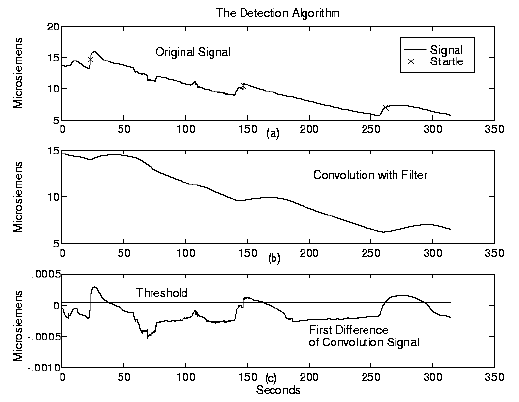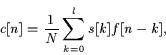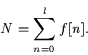Next: Experimental Validation Up: StartleCam: A Cybernetic Wearable Previous: The Skin Conductivity Response

# The Detection Algorithm

The detection algorithm processes the incoming signal from the skin conductance sensor using convolution with a matched filter, the first forward difference and a threshold comparison. The filter was chosen to match the rising arm of the startle response, as shown in Figure  2. In psychophysiological studies, the rising arm of the response has proved to be both more significant and less dependent on environmental variables than the decay  [SF90]. This rising arm filter is shorter than one capturing the entire response and reduces the calculations required for convolution sum in the detection algorithm. Using this filter also improves detection when the decay of the response is corrupted by a second startle. The digital filter, f[n], of length l (for the filter shown l=800), used for detection is a time-reversed rising edge of a typical startle response. The matched filter can be tailored to best represent the response of individual users, however the shape of the response is similar for many people and in this paper the typical response filter in Figure  2 was used for all subjects.To detect the startle response, the incoming signal from the skin conductivity sensor, s[n], is convolved with the time-reversed matched filter f[n], resulting in the convolution sum, c[n], according to the equation:(1)

where N is the normalization factor for the matched filter:(2)

The first forward difference, d[n], is then calculated for every point of the convolution sum:

 d[n]=c[n]-c[n-1]. (3)

When the value of the difference vector, d[n], changes from a value below a set threshold to a value above that threshold, the algorithm declares that a startle has been detected. A second startle will not be detected until the value of the difference vector drops below and then above the threshold. Also, the algorithm will not detect a startle in the first ten seconds of processing, allowing time for the buffer of video images to fill and the leading artifacts of the convolution sum to be cleared from the convolution buffer.Next: Experimental Validation Up: StartleCam: A Cybernetic Wearable Previous: The Skin Conductivity Response
Jennifer Healey - fenn@media.mit.edu
1999-02-12